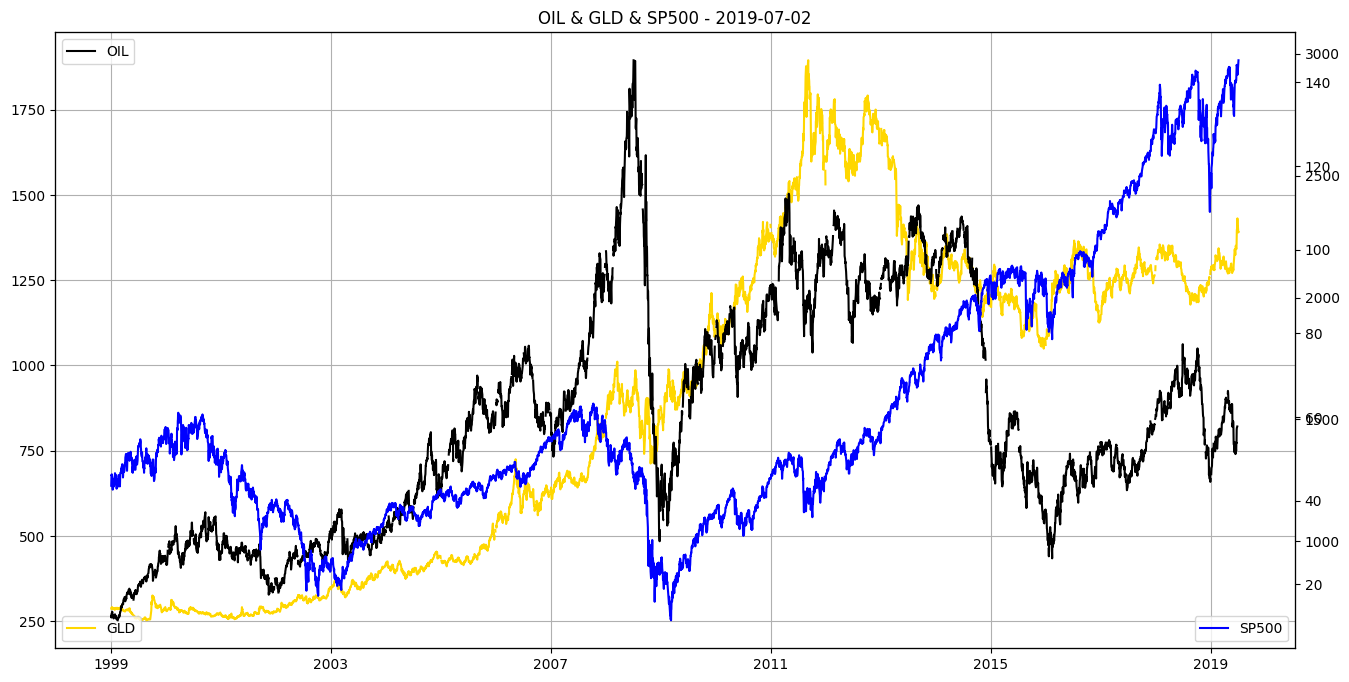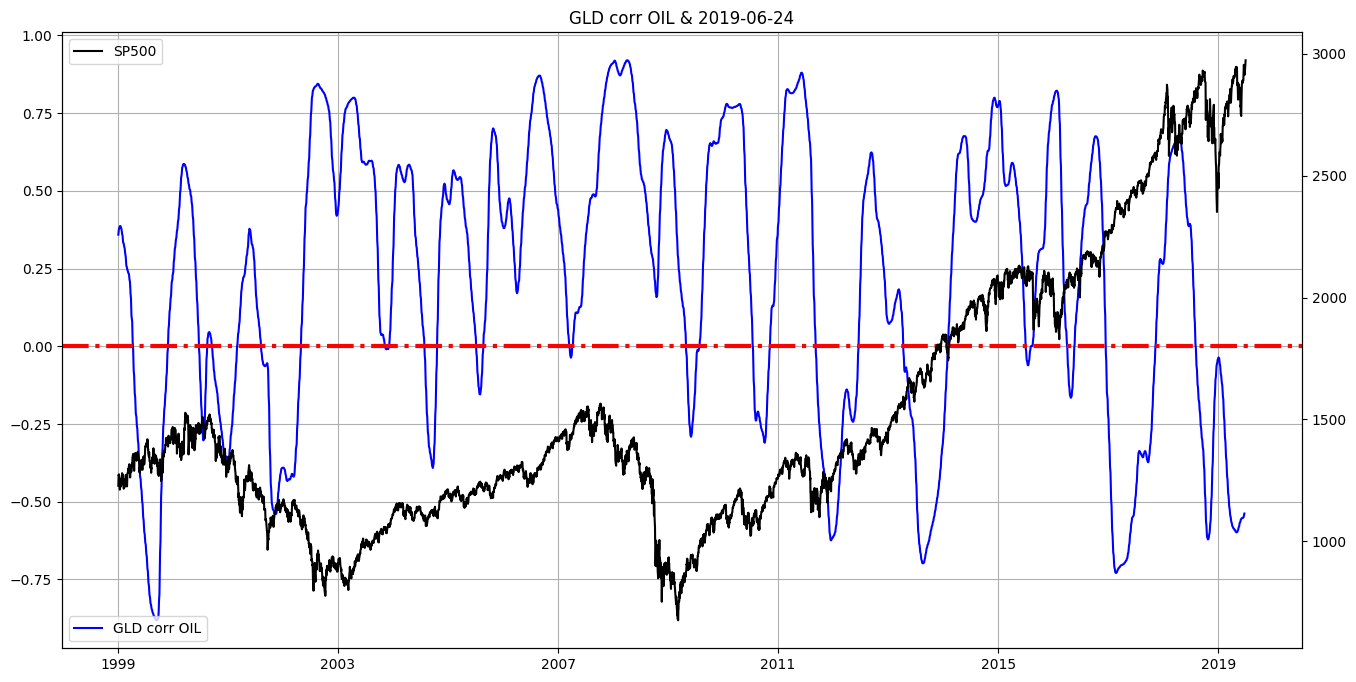My New Udemy Class: Fundamental Market Analysis with PythonFundamental Market Analysis with Python - Find Your Own Answers On What Is Going on in the Financial Markets

Hot off the Press!My New Book: "The Little Book of Fundamental Analysis: Hands-On Market Analysis with Python" is Out!

Grow Your Web Brand, Visibility & Traffic Organically5 Years of amunategui.github.Io and the Lessons I Learned from Growing My Online Community from the Ground Up

Introduction

The Oil and Gold Decouple! What does it mean? What happens when the price of oil moves in the opposite direction than gold? People say it's a bad omen when that happens.

Code

ViralML-Hands-On-Gold-Oil-Decouple
In :
from IPython.display import Image
Image(filename='viralml-book.png')
Out:In :
%matplotlib inline
import matplotlib
import matplotlib.pyplot as plt

import matplotlib as mpl
import matplotlib.dates as mdates
from mpl_finance import candlestick_ohlc
mpl.style.use('default')

import io, base64, os, json, re
import pandas as pd
import numpy as np
import datetime
import warnings
warnings.filterwarnings('ignore')
In :
path_to_market_data = '/Users/manuel/Documents/financial-research/market-report/2019-07-03/'

In :
# https://finance.yahoo.com/quote/%5EGSPC
sp500_df = pd.read_csv(path_to_market_data + '^GSPC.csv')
sp500_df['Date'] = pd.to_datetime(sp500_df['Date'])
sp500_df = sp500_df[['Date','Adj Close']]
sp500_df.columns = ['Date', 'SP500_Close']
print(np.min(sp500_df['Date'] ),np.max(sp500_df['Date'] ))
sp500_df = sp500_df.sort_values('Date', ascending=True) # sort in ascending date order
sp500_df.tail()
1950-01-03 00:00:00 2019-07-02 00:00:00
Out:
Date SP500_Close
17482 2019-06-26 2913.780029
17483 2019-06-27 2924.919922
17484 2019-06-28 2941.760010
17485 2019-07-01 2964.330078
17486 2019-07-02 2973.010010

Gold & OIL¶

Gold Price: London Fixing¶

In :
# https://www.quandl.com/data/LBMA/GOLD-Gold-Price-London-Fixing
gold_df = pd.read_csv(path_to_market_data + 'LBMA-GOLD.csv')
gold_df['Date'] = pd.to_datetime(gold_df['Date'])

gold_df = gold_df[['Date', 'USD (PM)']]
gold_df.columns = ['Date', 'GLD']
gold_df['GLD'] = pd.to_numeric(gold_df['GLD'], errors='coerce')

print(np.min(gold_df['Date'] ),np.max(gold_df['Date'] ))
gold_df = gold_df.sort_values('Date', ascending=True) # sort in ascending date order

gold_df['GLD_pct_change'] = gold_df['GLD'].pct_change()
gold_df.tail()
1968-01-02 00:00:00 2019-07-02 00:00:00
Out:
Date GLD GLD_pct_change
4 2019-06-26 1403.95 -0.019177
3 2019-06-27 1402.50 -0.001033
2 2019-06-28 1409.00 0.004635
1 2019-07-01 1390.10 -0.013414
0 2019-07-02 1391.05 0.000683
In :
# Crude Oil Prices: West Texas Intermediate (WTI)
# https://fred.stlouisfed.org/series/DCOILWTICO
oil_df = pd.read_csv(path_to_market_data + 'DCOILWTICO.csv')
oil_df['Date'] = pd.to_datetime(oil_df['DATE'])

oil_df = oil_df[['Date', 'DCOILWTICO']]
oil_df.columns = ['Date', 'OIL']
oil_df['OIL'] = pd.to_numeric(oil_df['OIL'], errors='coerce')

print(np.min(oil_df['Date'] ),np.max(oil_df['Date'] ))
oil_df = oil_df.sort_values('Date', ascending=True) # sort in ascending date order

oil_df['OIL_pct_change'] = oil_df['OIL'].pct_change()
oil_df.tail()
1986-01-02 00:00:00 2019-06-24 00:00:00
Out:
Date OIL OIL_pct_change
8728 2019-06-18 53.86 0.036966
8729 2019-06-19 53.74 -0.002228
8730 2019-06-20 56.88 0.058429
8731 2019-06-21 57.35 0.008263
8732 2019-06-24 57.73 0.006626

Visualize¶

In :
gold_df_tmp = gold_df.copy()
oil_df_tmp = oil_df.copy()
sp500_df_tmp = sp500_df.copy()

cut_off_date = '1999-01-01'
gold_df_tmp = gold_df_tmp[gold_df_tmp['Date'] >= cut_off_date]
oil_df_tmp = oil_df_tmp[oil_df_tmp['Date'] >= cut_off_date]
sp500_df_tmp = sp500_df_tmp[sp500_df_tmp['Date'] >= cut_off_date]

fig, ax = plt.subplots(figsize=(16, 8))
plt.plot(gold_df_tmp['Date'], gold_df_tmp['GLD'], label='GLD', color='gold')
plt.legend(loc='lower left')
plt.title('OIL & GLD & SP500 - ' + str(np.max(gold_df_tmp['Date']))[0:10])
plt.grid()

# Get second axis
ax2 = ax.twinx()
plt.plot(oil_df_tmp['Date'],  oil_df_tmp['OIL'], label='OIL',color='black')
plt.legend(loc='upper left')

# Get third axis
ax3 = ax.twinx()
plt.plot(sp500_df_tmp['Date'],  sp500_df_tmp['SP500_Close'], label='SP500',color='blue')
plt.legend(loc='lower right')
plt.show()Let's do a correlation on gold and oil to better understand the relationship¶

In :
# join data sets together
# join both datasets together
together = pd.merge(gold_df, oil_df, on= ['Date'], how='inner')

# last valid observation forward
together = together.fillna(method='ffill')

# drop NAs
together = together.dropna(axis=0)
together.tail()

together['corr'] = together['GLD'].rolling(200).corr(together['OIL'].rolling(200))
together['corr'] = together['corr'].rolling(10).mean()

together.tail()
Out:
Date GLD GLD_pct_change OIL OIL_pct_change corr
8456 2019-06-18 1341.35 0.000037 53.86 0.036966 -0.550647
8457 2019-06-19 1344.05 0.002013 53.74 -0.002228 -0.549177
8458 2019-06-20 1379.50 0.026376 56.88 0.058429 -0.546610
8459 2019-06-21 1397.15 0.012794 57.35 0.008263 -0.542959
8460 2019-06-24 1405.70 0.006120 57.73 0.006626 -0.538138
In :
together_tmp = together.copy()
sp500_df_tmp = sp500_df.copy()

together_tmp = together_tmp[together_tmp['Date'] >= cut_off_date]
sp500_df_tmp = sp500_df_tmp[sp500_df_tmp['Date'] >= cut_off_date]

fig, ax = plt.subplots(figsize=(16, 8))
plt.plot(together_tmp['Date'], together_tmp['corr'], label='GLD corr OIL', color='blue')
plt.legend(loc='lower left')
plt.title('GLD corr OIL & ' + str(np.max(together_tmp['Date']))[0:10])
plt.axhline(0, color='red', linewidth=3, linestyle='-.' )
plt.grid()

# Get second axis
ax2 = ax.twinx()
plt.plot(sp500_df_tmp['Date'],  sp500_df_tmp['SP500_Close'], label='SP500',color='black')
plt.legend(loc='upper left')
Out:
<matplotlib.legend.Legend at 0x11e88be48>Show Notes

(pardon typos and formatting -
these are the notes I use to make the videos)

The Oil and Gold Decouple! What does it mean? What happens when the price of oil moves in the opposite direction than gold? People say it's a bad omen when that happens.